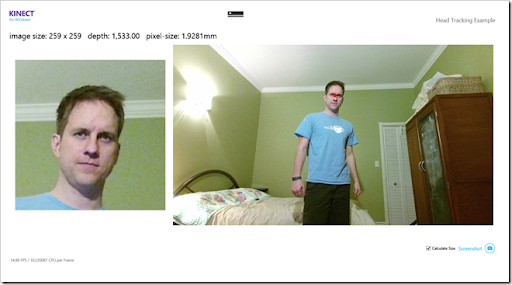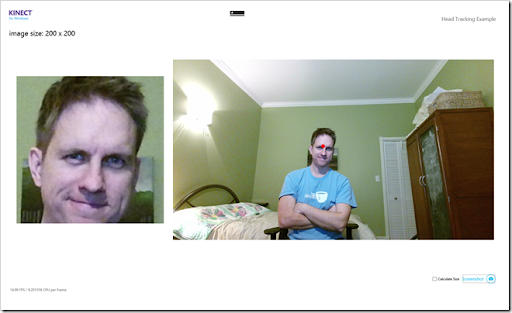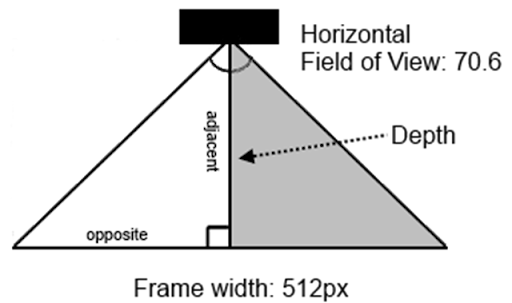## Wednesday, March 26, 2014

### Head Tracking with Kinect v2

This is yet another post in my series about the new Kinect using the November 2013 developer preview SDK. Today we’re going to have some fun by combining the color, depth and body data streams (mentioned in my last few posts, here, here and here) and some interesting math to create an image that magically tracks the user’s head.
This is an early preview of the new Kinect for Windows, so the device, software and documentation are all preliminary and subject to change.If you recall from my last post, I used the CoordinateMapper to translate the coordinates of the user’s joint information on top of the HD color image. The magic ingredient converts the Joint’s Position to a ColorSpacePoint.

```Joint headJoint = body.Joints[JointType.Head];

ColorSpacePoint colorSpacePoint =
If we take the X & Y coordinates from this ColorSpacePoint and the wonderful extension methods of the WriteableBitmapEx project, we can quickly create a cropped image of that joint.
```int x = (int)Math.Floor(colorSpacePoint.X + 0.5);
int y = (int)Math.Floor(colorSpacePoint.Y + 0.5);

int size = 200;

WriteableBitmap faceImage = _bmp.Crop(new Rect(x,y,size,size));```Wow, that was easy! Although this produces an image that accurately tracks my head, the approach is somewhat flawed as it doesn’t scale based on the user’s position from the camera: if you stand too close to the camera you’ll only see a portion of your face; Stand too far and you’ll see my face and torso. We can fix this by calculating the desired size of the image based on the depth of the joint. To do this, we’ll need to obtain a DepthSpacePoint for the Joint and a simple trigonometric formula…
The DepthSpacePoint by itself doesn’t contain the depth data. Instead, it contains the X & Y coordinates from the depth image which we can use to calculate the index in the array of depth data. I’ve outlined this in a previous post, but for convenience sake here’s that formula again:
```// get the depth image coordinates for the head
DepthSpacePoint depthPoint =

// use the x & y coordinates to locate the depth data
FrameDescription depthDesc = _sensor.DepthFrameSource.FrameDescription;
int depthX = (int)Math.Floor(depthPoint.X + 0.5);
int depthY = (int)Math.Floor(depthPoint.Y + 0.5);
int depthIndex = (depthY * depthDesc.Width) + depthX;

ushort depth = _depthData[depthIndex];```
To calculate the desired size of the image, we need to determine the width of the joint's pixel in millimeters. We do this using a blast from the past, our best friend from high-school trigonometry, Soh-Cah-Toa.Given that the Kinect’s Horizontal Field of View is 70.6°, we bisect this in half to form a right-angle triangle. We then take the depth value as the length of the adjacent side in millimeters. Our goal is to calculate the opposite side in millimeters, which we can accomplish using the TOA portion of the mnemonic:
```tan(0) = opposite / adjacent
Once we have the length of the opposite, we divide it by the number of pixels in the frame which gives us the length in millimeters for each pixel. The algorithm for calculating pixel width is shown here:
```private double CalculatePixelWidth(FrameDescription description, ushort depth)
{
// measure the size of the pixel
float hFov = description.HorizontalFieldOfView / 2;
float numPixels = description.Width / 2;

/* soh-cah-TOA
*
* TOA = tan(0) = O / A
*   T = tan( (horizontal FOV / 2) in radians )
*   O = (frame width / 2) in mm
*   A = depth in mm
*
*   O = A * T
*/

double T = Math.Tan((Math.PI * 180) / hFov);
double pixelWidth = T * depth;

return pixelWidth / numPixels;
}```
Now that we know the length of each pixel, we can adjust the size of our head-tracking image to be a consistent “length”. The dimensions of the image will change as I move but the amount of space around my head remains consistent. The following calculates a 50 cm (~19”) image around the tracked position of my head:
```double imageSize = 500 / CalculatePixelWidth(depthDesc, depth);

int x = (int)(Math.Floor(colorPoint.X + 0.5) - (imageSize / 2));
int y = (int)(Math.Floor(colorPoint.Y + 0.5) - (imageSize / 2));

WriteableBitmap faceImage = _bmp.Crop(new Rect(x,y, imageSize, imageSize));```
Happy Coding.

Kurt S said...

Good ol' Soh-Cah-Toa

Interesting post! The youtube video helped me understand the problem at hand. The solution seems to work really well.

Looks like the 2.0 SDK has much better/quicker skeleton tracking compared to 1.x. Does it track skeletons when the user walks by (perpendicular to the sensor)?

-Kurt

kinectJockey said...

Hi bryan, Its a very good and simple tutorial to understand ,Kudos to that.

My query is.Can the colorstream be centered according to the user , like the head image which align always at the center. how can the whole body be aligned at the center.in the colorstream,. Thanks in advance

bryan said...

@kinectJockey I think you could easily adjust this sample to center on the user by changing the joint to MidSpine instead of the Head joint. The joints are listed here: https://msdn.microsoft.com/en-us/library/windowspreview.kinect.jointtype.aspx

You would likely want to capture about 100cm on either side of the joint.